Courses

# Practice Doc: CAT 2020 For Quant (Slot - 1) Notes | EduRev

## CAT : Practice Doc: CAT 2020 For Quant (Slot - 1) Notes | EduRev

The document Practice Doc: CAT 2020 For Quant (Slot - 1) Notes | EduRev is a part of CAT category.
All you need of CAT at this link: CAT

Q.1. How many 3-digit numbers are there, for which the product of their digits is more than 2 but less than 7?
Ans: 21
Solution: The product of the digits of the three-digit numbers should be more than 2 and less than 7. Hence the possible numbers are as follows.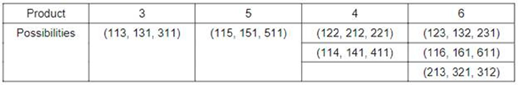Hence there are a total of 21 possibilities.

Q.2. Veeru invested Rs 10000 at 5% simple annual interest, and exactly after two years, Joy invested Rs 8000 at 10% simple annual interest. How many years after Veeru’s investment, will their balances, i.e., principal plus accumulated interest, be equal?
Ans: 12
Solution: Let after n years both the sums amount to the equal amounts.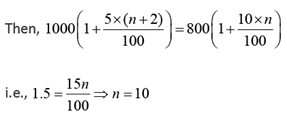Hence 12 years after veeru invested their balances will be equal

Q.3. An alloy is prepared by mixing three metals A, B and C in the proportion 3: 4: 7 by volume. Weights of the same volume of the metals A, B and C are in the ratio 5: 2: 6. In 130 kg of the alloy, the weight, in kg, of the metal C is
(a) 70
(b) 96
(c) 48
(d) 84
Ans: d
Solution: Required weight of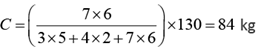Q.4. On a rectangular metal sheet of area 135 sq in, a circle is painted such that the circle touches two opposite sides. If the area of the sheet left unpainted is two-thirds of the painted area then the perimeter of the rectangle in inches is
(a)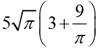(b)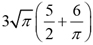(c)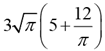(d)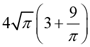Ans: c
Solution: Let the length and the breadth of the rectangle be l and b respectively.
As the circle touches the two opposite sides, its diameter will be same as the breadth of the rectangle. Given, lb = 135 and lb = π(b/2)2  =2/3 x π(b/2)2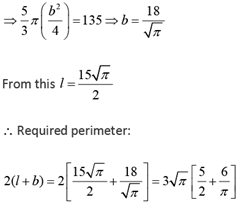Q.5. If log4 5 = (log4y) (log6√5), then y equals
Ans: 36
Solution: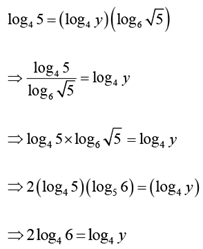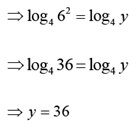Q.6. Two persons are walking beside a railway track at respective speeds of 2 and 4 km per hour in the same direction. A train came from behind them and crossed them in 90 and 100 seconds, respectively. The time, in seconds, taken by the train to cross an electric post is nearest to
(a) 87
(b) 82
(c) 75
(d) 78

Ans: b
Solution: Let the length of the train be l and its speed be s. Given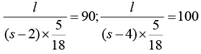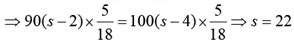∴ Length of the train =500 m.
Hence the required time to cross a lamp post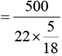i.e., 81.81 (or) 82 sec.

Q.7. The number of real-valued solutions of the equation 2x + 2- x = 2 - (x - 2)2 is
(a) infinite
(b) 1
(c) 0
(d) 2
Ans: c
Solution: 2x + 2-x = 2 - (x - 2)2
The minimum value of 2x + 2-x is 2 when x = 0
But x = 0; 2 - (x - 2)2 = - 2
The maximum value of 2 - (x - 2)is 2 when x = 2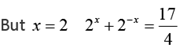Hence there is no value of x, 2x + 2-x = 2 - (x - 2)2
The number of solutions is 0

Q.8. If y is a negative number such that 2y2 log3 5 = 5log2 3, then y equals
(a) log2 (1/3)
(b) - log2 (1/3)
(c) log2 (1/5)
(d) - log2 (1/5)
Ans:
a
Solution: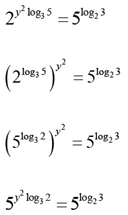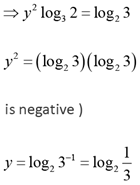Q.9. How many distinct positive integer-valued solutions exist to the equation (x2 - 7x + 11) (x2 - 13x + 42) = 1?
(a) 6
(b) 8
(c) 2
(d) 4
Ans: a
Solution: (x2 - 7x + 11) (x2 - 13x + 42) = 1
We know if ab = 1
⇒ a = 1 and b is any number
or a = - 1 and b is even
a > 0 and b is 0
case 1: x-13x + 42 = 0 ⇒ x = 6, 7
case 2 : x2 - 7x +11 = 1 ⇒ x2 - 7x +10 = 0 ⇒ x = 2 or 5
case 3: x2 - 7x +11 = -1 ⇒ x2 - 7x +12 = 0
⇒ x = 4 or 3
Hence number of solutions are 6

Q.10. A person spent Rs 50000 to purchase a desktop computer and a laptop computer. He sold the desktop at 20% profit and the laptop at 10% loss. If overall he made a 2% profit then the purchase price, in rupees, of the desktop is
Ans:
20000
Solution: Using Alligation Rule, the ratio of cost prices of desktop and laptop will be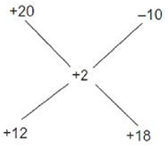i.e., 2: 3
∴ The cost of desktop = 2/5 x 50000 i.e., ? 20,000

Q.11. The area of the region satisfying the inequalities |x| - y ≤ 1, y ≥ 0 and y ≤ 1 is
Ans: 3
Solution: The graph of |x| - y ≤ 1, y ≥ 0 and y ≤ 1 is as follows: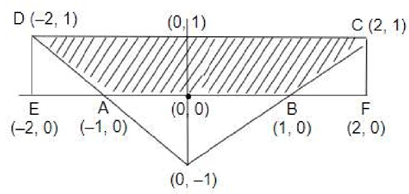Area of ABCD = Area of EFCD - Area of EAD - Area of BFC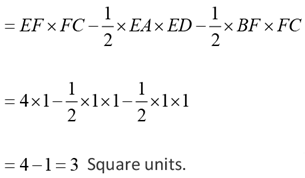Q.12. A straight road connects points A and B. Car 1 travels from A to B and Car 2 travels from B to A, both leaving at the same time. After meeting each other, they take 45 minutes and 20 minutes, respectively, to complete their journeys. If Car 1 travels at the speed of 60 km/hr, then the speed of Car 2, in km/hr, is
(a) 90
(b) 100
(c) 80
(d) 70
Ans:
a
Solution: In this particular case, we know Ratio of speeds =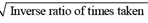C1 : C2 = √20 : 45 i.e., 2: 3
As the speed of Car C1 is 60 kmph, the speed of Car C2 is 90 kmph

Q.13. Among 100 students, x1 have birthdays in January, x2 have birthdays in February, and so on. If x0 = max (x1, x2,....x12) then the smallest possible value of x0 is
(a) 9
(b) 10
(c) 8
(d) 12
Ans:
a
Solution: Given x0 = (x1, x2, ..........x12)
If x1 = x2 = x3 = x4 = 9; x5 = x6 = .....x12 = 8
∴ x0 =  max(9, 9, 9, 9, 8, 8.....8)
The minimum value if x0 is 9.

Q.14. The mean of all 4 -digit even natural numbers of the form 'aabb', where a >0, is
(a) 5050
(b) 4466
(c) 5544
(d) 4864
Ans:
c
Solution: The sum of possible even digit numbers in the form aabb is 1100 + 1122 + 1144 + 1166 + 1188 + 2200 + 2222 + 2288 + ¼.9900 + 9922 + 9988 i.e. (45 numbers)
⇒ 1100 + 1100 + 1100 + 1100 + 1100 + 22 + 44 + 66 + 88 + 2200 + 2200 + 2200 + 2200 + 2200
+22 + 44 + 66 + 88 +… + 9900 + 9900 + 9900 + 990 + 9900 + 22 + 44 + 66 + 88
⇒ 5(1100 + 2200 + ¼.9900) + 9(22 + 44 + 66 + 88)5 x 1100(1 + 2 + ..... 9) + 9 x 22(1 + 2 + 3 + 4)
⇒ 5500(45) + 45 x 44 = 45(5544)
Hence mean = 5544

Q.15. Leaving home at the same time, Amal reaches office at 10: 15 am if he travels at 8 km/hr, and at 9: 40 am if he travels at 15 km/hr. Leaving home at 9: 10 am, at what speed, in km/hr, must he travel so as to reach office exactly at 10 am?
(a) 13
(b) 14
(c) 12
(d) 11
Ans: c
Solution: Let the speed in the first two cases be s and the distance be "d'. Given,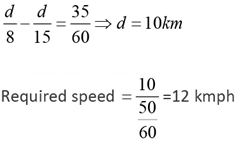Q.16. A train travelled at one-thirds of its usual speed, and hence reached the destination 30 minutes after the scheduled time. On its return journey, the train initially travelled at its usual speed for 5 minutes but then stopped for 4 minutes for an emergency. The percentage by which the train must now increase its usual speed so as to reach the destination at the scheduled time, is nearest to
(a) 58
(b) 67
(c) 61
(d) 50
Ans: b
Solution: Let the usual speed of the train be s and time taken at that speed be 't'.
Given by travelling at s/3, it reached 30 min late. Hence the usual time:
Distance travelled = s x t
Distance travelled in the first 5min = s x t / 3. D
Distance to be travelled in the last 6min = 2 st / 3
Required speed to cover that distance on time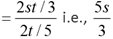Hence the percentage increase in its speed = (2 / 3) x 100 i.e., 661/2% or 67%

Q.17.  If x = ( 4096)7 + 4√3, then which of the following equals 64?
(a)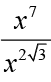(b)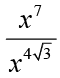(c)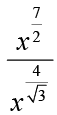(d)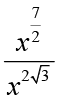Ans: d
Solution: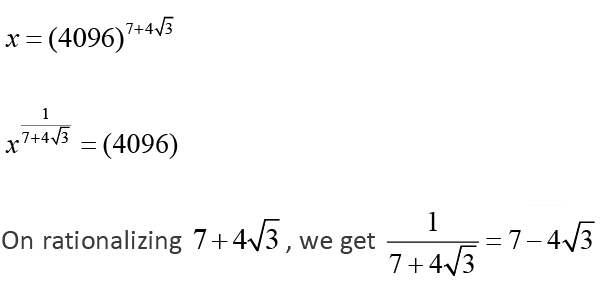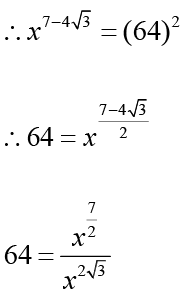Q.18. If f (5 + x) = f (5 - x) for every real x, and f(x) = 0 has four distinct real roots, then the sum of these roots is
(a) 0
(b) 40
(c) 10
(d) 20
Ans:
d
Solution: Given f (5 + x) = f (5 - x)
Put x = x - 5
f (x) = f (10 - x)
∴ Let a, b be two roots of f(x) = 0, then 10 - a,10 - b are also roots of f (x) = 0
∴ Hence sum of the roots = a + b + 10 - a + 10 - b = 20

Q.19. If a, b and c are positive integers such that ab = 432, bc = 96 and c < 9, then the smallest possible value of a + b + c is
(a) 56
(b) 59
(c) 49
(d) 46
Ans:
d
Solution: Given ab = 432, bc = 96 and c < 9
To find the minimum value for a + b + c, the two larger numbers should be as close as possible.
The closest combination whose product is 432 is 18 x 24 . For b = 24, we get c = 4 and a = 18.
Hence the least value for a + b + c = 46.

Q.20. In a group of people, 28% of the members are young while the rest are old. If 65% of the members are literates, and 25% of the literates are young, then the percentage of old people among the illiterates is nearest to
(a) 62
(b) 55
(c) 66
(d) 59

Ans: c
Solution: Let the number of persons in the group be 100.
Then the people who are young = 28
Ans the number of literate persons = 65
Number of literates who are young = 25% of 65 = 16.25
∴ Required answer = 48.75/72 x 100 = 66

Q.21. A circle is inscribed in a thombus with diagonals 12 cm and 16 cm. The ratio of the area of circle to the area of rhombus is
(a) 5π/18
(b) 6π/25
(c) 3π/25
(d) 2π/15
Ans: b
Solution: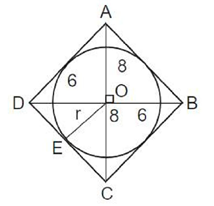Given the circle is inscribed in the rhombus of diagonals 12 and 16. Let O be the point of intersection of the diagonals of the rhombus. Then, OE (radius) ⊥ DC.
Also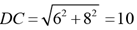As area of ΔODC should be the same, we have, 1/2 x 6 x 8 = 1/2 x OE x 10
⇒ OE = 4.8
∴ Required ratio of areas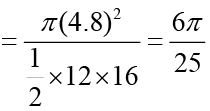Q.22. A gentleman decided to treat a few children in the following manner. He gives half of his total stock of toffees and one extra to the first child, and then the half of the remaining stock along with one extra to the second and continues giving away in this fashion. His total stock exhausts after he takes care of 5 children. How many toffees were there in his stock initially?
Ans: 62
Solution: Given that the person is left with no toffees after distributing them to the fifth student.
Also given that to each student the person gave one more than half the number of toffees at that stage.
For these types of problems, better we go for backward calculation. If the person had not given 1 extra toffee, he would have left with that toffee.
This represents that he had 2 toffees at that stage. In the previous stage i.e in 4th stage he should have (2 +1) x 2 i.e 6 toffees In the third stage, he should have (6 + 1) x 2 i.e 14 toffees.
In the second stage, he should have (14 + 1) x 2 i.e 30 toffees. In the first stage, he should have (30 + 1) x 2 i.e 62 toffees. Hence he initially had 62 toffees.

Q.23. Let A, B and C be three positive integers such that the sum of A and the mean of B and C is 5. In addition, the sum of B and the mean of A and C is 7. Then the sum of A and B is
(a) 6
(b) 5
(c) 7
(d) 4
Ans: a
Solution: Given A, B and C are positive integers such that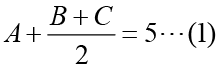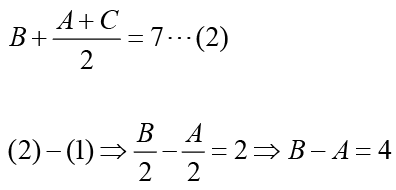The least value for A = 1 in which case B = 5.
Hence A + B = 6

Q.24. A solution, of volume 40 litres, has dye and water in the proportion 2 : 3. Water is added to the solution to change this proportion to 2 : 5. If one-fourths of this diluted solution is taken out, how many litres of dye must be added to the remaining solution to bring the proportion back to 2 : 3?
Ans: 8
Solution: Original quantity of dye and water in the original solution i.e., 16 litres (i.e. = 40 x 2/5) and 24 litres (i.e. = 40 - 16)
Quantity of water added = 16 litres (As 1 part = 8 litres). Quantity of dye and water removed = 1/4 x 2/7(5/6) i.e., 4 litres and 1/4 x 5/7 x (56) i.e., 10 litres. Final quantity of dye and water is 12 litres and 30 litres.
∴ Quantity of dye to be added to make the ratio of dye and water again 2: 3 i.e., 8 litres.

Q.25. The number of distinct real roots of the equation (x + 1/x)2 - 3(x + 1/x) +2 = 0 equals
Ans:
1
Solution: Let x + 1/x = a
The given equation becomes, a2 - 3a + 2 = 0 a = 2 or 1 i.e x + 1/x = 2 or x + 1/x = 1
since x is real,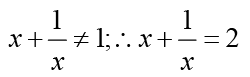∴ The number of solutions = 1

Q.26. A solid right circular cone of height 27 cm is cut into two pieces along a plane parallel to its base at a height of 18 cm from the base. If the difference in volume of the two pieces is 225 cc, the volume, in cc, of the original cone is
(a) 232
(b) 256
(c) 264
(d) 243
Ans: d
Solution: As the cone is cut at one-third height from the top (the vertex), the total volume is proportional to the cubes of the heights of the two parts.
Ratio of volumes two parts = (1/3)3 : 13 = 1 : 27
Hence the bottom part will have volume of 27 - 1 i.e., 26 parts.
Given ( 26 - 1) i.e., 25 parts -225 cc.
Hence the required answer is 27 parts = 27 x 225/25 = 243cc.

Offer running on EduRev: Apply code STAYHOME200 to get INR 200 off on our premium plan EduRev Infinity!

,

,

,

,

,

,

,

,

,

,

,

,

,

,

,

,

,

,

,

,

,

;